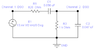# Electrical eng, my vectors for KVL and KCL aren't adding up - no errors in math

Yarnzorrr
I've been asked to prove KVL and KCL using this circuit, but my vectors don't add up.
ei Vr1+ Vr2 + Vc1+Vc2 ≠ Vtotal, same goes for Current, but I at least got the correct angle for the current
Tried using vector addition, using addition of the vectors in complex form and even tried using this http://www.1728.org/vectors.htm vector addition calculator

I know my calculation are correct, I got my lab supervisor to check them.

These are the values that I've got for my series parallel RC circuit (attached a photo of the circuit diagram)

Voltage (V)
R1 1.60< 49.97°
C1 2.78 < - 40.03°
R1 1.68 <19.4°
C2 1.68 <19.4°
Total 4.75< 0°

Currant (mA)
R1 1.956 < 49.97°
C1 1.956 < 49.97°
R2 1.68<19.4°
C2 0.995< 109.4°
Total 1.956 < 49.9°

Impedance (Ω)
R1 820 < 0°
C1 1421< -90°
R2 1000 < 0°
C2 1693< -90°
Total 2427.6 < - 49.9°

#### Attachments

•Screen Shot 2012-09-27 at 4.38.07 PM.png
3.4 KB · Views: 395

Mentor
I've been asked to prove KVL and KCL using this circuit, but my vectors don't add up.
ei Vr1+ Vr2 + Vc1+Vc2 ≠ Vtotal, same goes for Current, but I at least got the correct angle for the current
Tried using vector addition, using addition of the vectors in complex form and even tried using this http://www.1728.org/vectors.htm vector addition calculator

I know my calculation are correct, I got my lab supervisor to check them.

These are the values that I've got for my series parallel RC circuit (attached a photo of the circuit diagram)

Voltage (V)
R1 1.60< 49.97°
C1 2.78 < - 40.03°
R1 1.68 <19.4°
C2 1.68 <19.4°
Total 4.75< 0°

Currant (mA)
R1 1.956 < 49.97°
C1 1.956 < 49.97°
R2 1.68<19.4°
C2 0.995< 109.4°
Total 1.956 < 49.9°

Impedance (Ω)
R1 820 < 0°
C1 1421< -90°
R2 1000 < 0°
C2 1693< -90°
Total 2427.6 < - 49.9°

Can you show your analysis steps? I can see issues with your result values, but without seeing how you got there it's difficult to know how to help you.

Yarnzorrr
Forgot to mention that the AC voltage input value on the diagram is wrong, its actually 4.75 < 0° sorry!

first found Z with:

Ztotal = (R2*Xc2)/(√(R2^2+Xc2^2) + R1 + Xc1

Where
R2 = 1000
Xc2 = -j 1693
R1 = 820
Xc2 = -j 1421

break it down to polar form to get

861 < 30.57° + 820 <0° + 1421 <-90° = 2427.6 < - 49.97°

Then made a table and using Ohm's Law got the following (attached)

#### Attachments

Yarnzorrr
and to get the current through R2 and Xc2:

Itotal * (R2/(-jxc2+R2) = 1.956 < 49.97° * (1000 /-j1693+1000)

then to get IXc swapped the value for R2 on the denominator for Xc